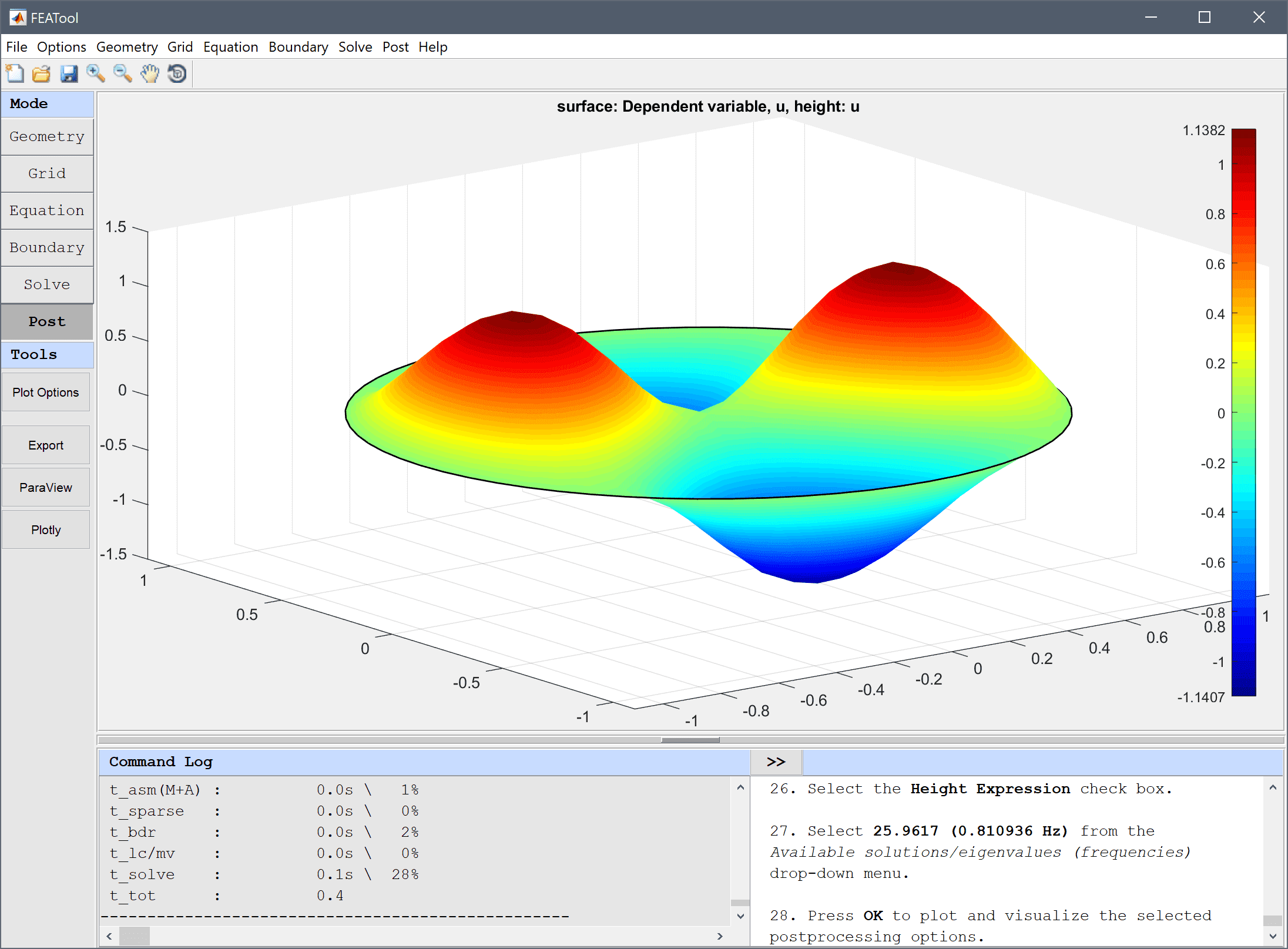# Vibrations of a Circular Membrane## Model Data

Type: classic pde

Physics Modes: poisson equation

Keywords: resonance frequencies validation

This example computes the the vibration modes, eigenvalues, and frequencies for a circular drum or membrane. The membrane is modeled by the unit circle and assumed to be attached to a rigid frame. The Poisson PDE equation is used with the Eigenvalue solver to compute the solution.

This model is available as an automated tutorial by selecting Model Examples and Tutorials… > Classic PDE > Vibrations of a Circular Membrane from the File menu. Or alternatively, follow the linked step-by-step instructions.